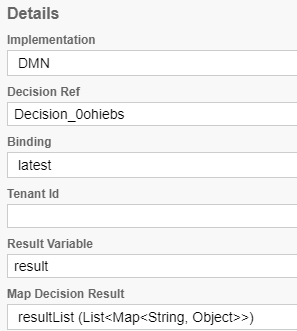# How to parse the decision result on the "input/output" tab of a business rule task?

Hello,

My decision table (hit policy collect) can return back multiple rows (rules), that each of them has two values (columns).

For example, in case that two rows of the DMN table match,
I have a result like the following:

``````[{"assignee":"smith","group":"IT"},{"assignee":"tomas","group":"HR"}]
``````

Returning back to the properties panel of the business rule task, the “General” tab, looks like this:Further, I need in the “Input/Output” tab to go through the list of results, picking the “group” value from each row. I want to do this by using javascript.

Something like the following does not work:

What do I need to change inside my js code?

``````var i;
(for i=0;i<result.length;i++){
candidateGroup=result[i].group;
}
``````

After I will use the candidateGroup in the parameter “Candidate Groups” of a user task.
So, I will use something like this: \${candidateGroup}

Thank you,
Smith.

Hi Smith,

So if I understand your issue correctly, you want to transform this snippet:

``````[{"assignee":"smith","group":"IT"},{"assignee":"tomas","group":"HR"}]
``````

into:

``````["IT", "HR"]
``````

If that’s correct, the following snippet should do the trick:

``````var i, output = [];
for (i = 0; i < result.length; i++) {
output.push(result[i].group);
}
output
``````

Please try this out and let me know if that’s what solves your problem.

``````output
I tried this on a simple script task and it seems that instead of plain `output` you may need to convert it first to a proper java type: `Java.to(output, 'java.lang.String[]')`.